Next: Frequency properties. Up: Results Previous: Spectrum

## Wave-amplitude probability density function and its moments

Now we consider the PDF of amplitudes for which predictions where made recently within the WT approach. To measure the PDF of amplitudes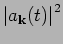, we set two radial regions in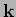-space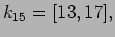and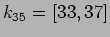. These regions were inside the inertial range and had well mixed phases and amplitudes since the experiment was done after performing 2000 rotations of the peak mode. We looked at the time-span of approximately 855 rotations of modes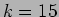or 1230 rotations of modes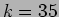and collected amplitudes of all modes from these three regions. The number of amplitudes collected was over 1.1 million in region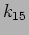and over 2.1 million in region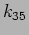.

 [width=10cm]akpdf15.eps
 [width=10cm]akpdf35.eps
Figure 3 shows a log-plot of the PDF the amplitude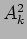inand an exponential fit of its low-amplitude part. One can see intermittency, i.e., an anomalously large probability of strong waves. We can also see that this discrepancy from Gaussianity happens in the tail, i.e. well below the mean amplitude value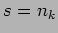. While the PDF tail in not long enough for drawing decisive conclusions about realization of the theoretically predicted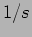scaling, it certainly gives a conclusive evidence that the probabilities of large amplitudes are orders of magnitude higher than in Gaussian turbulence. Figure 4 shows the PDF ofin. We can see some non-Gaussianity inas well, although much less than in. Similar conclusion that the gravity wave turbulence is more intermittent at low rather than high wavenumbers was reached on the basis of numerical simulations in .

Deviations from Gaussianity can be also seen in figure 5 which shows ratio of the moments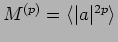to their values in Gaussian turbulence,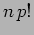. Again, we can see that such deviations are greater at the small-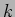part of the inertial range.

 [width=10cm]moments.epsNext: Frequency properties. Up: Results Previous: Spectrum
Dr Yuri V Lvov 2007-01-16